1641340800

# Swifter

## swifter

A package which efficiently applies any function to a pandas dataframe or series in the fastest available manner.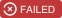## Documentation

To know about latest improvements, please check the changelog.

Further documentations on swifter is available here.

Check out the examples notebook, along with the speed benchmark notebook. The benchmarks are created using the library perfplot.

## Installation:

``````\$ pip install -U pandas # upgrade pandas
\$ pip install swifter # first time installation
\$ pip install swifter[modin-ray] # first time installation including modin[ray]
\$ pip install swifter[modin-dask] # first time installation including modin[dask]

``````

alternatively, to install on Anaconda:

``````conda install -c conda-forge swifter
``````

...after installing, import `swifter` into your code along with `pandas` using:

``````import pandas as pd
import swifter
``````

...alternatively, `swifter` can be used with `modin` dataframes in the same manner:

``````import modin.pandas as pd
import swifter
``````

NOTE: if you import swifter before modin, you will have to additionally register modin: `swifter.register_modin()`

## Easy to use

``````df = pd.DataFrame({'x': [1, 2, 3, 4], 'y': [5, 6, 7, 8]})

# runs on single core
df['x2'] = df['x'].apply(lambda x: x**2)
# runs on multiple cores
df['x2'] = df['x'].swifter.apply(lambda x: x**2)

# use swifter apply on whole dataframe
df['agg'] = df.swifter.apply(lambda x: x.sum() - x.min())

# use swifter apply on specific columns
df['outCol'] = df[['inCol1', 'inCol2']].swifter.apply(my_func)
df['outCol'] = df[['inCol1', 'inCol2', 'inCol3']].swifter.apply(my_func,
positional_arg, keyword_arg=keyword_argval)
``````

## Vectorizes your function, when possible## When vectorization is not possible, automatically decides which is faster: to use dask parallel processing or a simple pandas apply## Notes

The function is documented in the .py file. In Jupyter Notebooks, you can see the docs by pressing Shift+Tab(x3). Also, check out the complete documentation here along with the changelog.

Please upgrade your version of pandas, as the pandas extension api used in this module is a recent addition to pandas.

Import modin before importing swifter, if you wish to use modin with swifter. Otherwise, use `swifter.register_modin()` to access it.

Do not use swifter to apply a function that modifies external variables. Under the hood, swifter does sample applies to optimize performance. These sample applies will modify the external variable in addition to the final apply. Thus, you will end up with an erroneously modified external variable.

It is advised to disable the progress bar if calling swifter from a forked process as the progress bar may get confused between various multiprocessing modules.

If swifter return is different than pandas try explicitly casting type e.g.: `df.swifter.apply(lambda x: float(np.angle(x)))`

Author: jmcarpenter2
Source Code: https://github.com/jmcarpenter2/swifter

## Buddha Community1641340800

## swifter

A package which efficiently applies any function to a pandas dataframe or series in the fastest available manner.## Documentation

To know about latest improvements, please check the changelog.

Further documentations on swifter is available here.

Check out the examples notebook, along with the speed benchmark notebook. The benchmarks are created using the library perfplot.

## Installation:

``````\$ pip install -U pandas # upgrade pandas
\$ pip install swifter # first time installation
\$ pip install swifter[modin-ray] # first time installation including modin[ray]
\$ pip install swifter[modin-dask] # first time installation including modin[dask]

``````

alternatively, to install on Anaconda:

``````conda install -c conda-forge swifter
``````

...after installing, import `swifter` into your code along with `pandas` using:

``````import pandas as pd
import swifter
``````

...alternatively, `swifter` can be used with `modin` dataframes in the same manner:

``````import modin.pandas as pd
import swifter
``````

NOTE: if you import swifter before modin, you will have to additionally register modin: `swifter.register_modin()`

## Easy to use

``````df = pd.DataFrame({'x': [1, 2, 3, 4], 'y': [5, 6, 7, 8]})

# runs on single core
df['x2'] = df['x'].apply(lambda x: x**2)
# runs on multiple cores
df['x2'] = df['x'].swifter.apply(lambda x: x**2)

# use swifter apply on whole dataframe
df['agg'] = df.swifter.apply(lambda x: x.sum() - x.min())

# use swifter apply on specific columns
df['outCol'] = df[['inCol1', 'inCol2']].swifter.apply(my_func)
df['outCol'] = df[['inCol1', 'inCol2', 'inCol3']].swifter.apply(my_func,
positional_arg, keyword_arg=keyword_argval)
``````

## Vectorizes your function, when possible## When vectorization is not possible, automatically decides which is faster: to use dask parallel processing or a simple pandas apply## Notes

The function is documented in the .py file. In Jupyter Notebooks, you can see the docs by pressing Shift+Tab(x3). Also, check out the complete documentation here along with the changelog.

Please upgrade your version of pandas, as the pandas extension api used in this module is a recent addition to pandas.

Import modin before importing swifter, if you wish to use modin with swifter. Otherwise, use `swifter.register_modin()` to access it.

Do not use swifter to apply a function that modifies external variables. Under the hood, swifter does sample applies to optimize performance. These sample applies will modify the external variable in addition to the final apply. Thus, you will end up with an erroneously modified external variable.

It is advised to disable the progress bar if calling swifter from a forked process as the progress bar may get confused between various multiprocessing modules.

If swifter return is different than pandas try explicitly casting type e.g.: `df.swifter.apply(lambda x: float(np.angle(x)))`

Author: jmcarpenter2
Source Code: https://github.com/jmcarpenter2/swifter1593931740

## Speed up your Pandas Processing with Swifter

As a Pythonist Data Scientist, your daily job would involve a lot of data processing and feature engineering using the Pandas package. From analyzing data to create a new feature to gain insight, forcing you to execute many different codes repeatedly. The problem is the bigger your data, the longer time to finish running each line of code.

In this article, I want to show you a simple package to speed up your Pandas processing called Swifter. Let’s just get started.

# Swifter

Swifter is a package that tries to efficiently apply any function to a Pandas Data Frame or Series object in the quickest available method. It is integrated with the Pandas object so that we would use this package only with a Pandas object such as Data Frame or Series.

Let’s try to see Swifter in action. For preparation, we need to install the Swifter package.

``````#Installing Swifter via Pip
pip install swifter

#or via conda
conda install -c conda-forge swifter
``````

In case you have not possessed the latest Pandas package, it is suggested to update the package into the newest version. This is because Pandas extension api used in the Swifter module is a recent addition to pandas.

``````#Update the Pandas package via pip
pip install -U pandas

#or via conda
conda update pandas
``````

When all the required packages ready, we could proceed to try Swifter. In this article, I would use the Reddit comment dataset from Kaggle. From here, we import all the packages to our notebook and read the dataset from CSV as usual.

``````#Import the package
import pandas as pd
import swifter

``````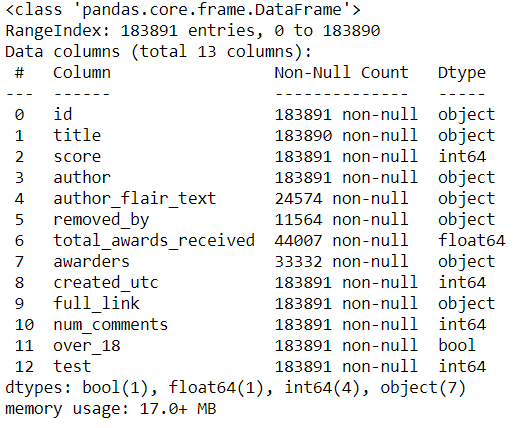This is our dataset. Now, let’s say I want to multiply the score by two and subtract the score by one (This is just a random equation I used here). Then I would put it in another column. In this case, I could use the apply function from the Pandas object attribute.

``````%time df['score_2_subs'] = df['score'].apply(lambda x: x/2 -1)
``````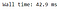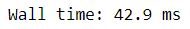Pandas apply Execution Time

The time to execute the function to each data takes around 42.9 ms for an apply attribute by Pandas. This time, we would use Swifter and see how much time it take to execute the function.

``````#When we importing the Swifter package, it would integrated with Pandas package and we could use functional attribute from Pandas such as apply

%time df['score_2_swift'] = df['score'].swifter.apply(lambda x: x/2 - 1)
``````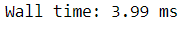Swifter apply Execution Time

As we can see above, Swifter processes the data way faster compared to the normal Pandas apply function.

# Vectorized Function for Swifter

From the documentation, it is stated that Swifter could apply function a hundred times faster than Pandas function. This, however, only applied if we are using a vectorized form of function.

Let’s say I create a function that evaluates the num_comments and score variable. When the comment count is zero, I will double the score. While it’s not, the score would stay the same. Then I would create a new column based on that.

``````def scoring_comment(x):
if x['num_comments'] == 0:
return x['score'] *2
else:
return x['score']
#Trying applying the function using Pandas apply
%time df['score_comment'] = df[['score','num_comments']].apply(scoring_comment, axis =1)
``````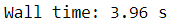Pandas apply with non-vectorized function Execution Time

It takes around 3.96 seconds to execute the function. Let’s see the performance if we are using Swifter.

``````%time df['score_comment_swift'] = df[['score', 'num_comments']].swifter.apply(scoring_comment, axis =1)
``````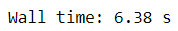Swifter apply with non-vectorized function Execution Time

As we can see above, it takes much longer using Swifter compared to the regular Pandas apply function. This is because Swifter with non-vectorized function would implement dask parallel processing, not relying on the Swifter processing itself. So, how is the performance if we change the function to the vectorized function? Let’s try it.

``````Import numpy as np

#Using np.where to implement vectorized function
def scoring_comment_vectorized(x):
return np.where(x['num_comments'] ==0, x['score']*2, x['score'])
#Trying using the normal Pandas apply
%time df['score_comment_vectorized'] = df[['score', 'num_comments']].apply(scoring_comment_vectorized, axis =1)
``````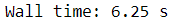Pandas apply with vectorized function Execution Time

It takes around 6.25 seconds using the normal apply function to execute our vectorized function. Let’s see the performance using Swifter.

``````%time df['score_comment_vectorized_swift'] = df[['score', 'num_comments']].swifter.apply(scoring_comment_vectorized, axis =1)
``````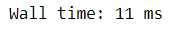Swifter apply with vectorized function Execution Time

The execution time now takes only 11 ms with the vectorized function, which saves so much time compared to the normal apply function. This is why it is advisable to use the vectorized function when we are processing data with Swifter.

If you want to keep track of the execution time that just happen, I will give you the overall summary in the table below.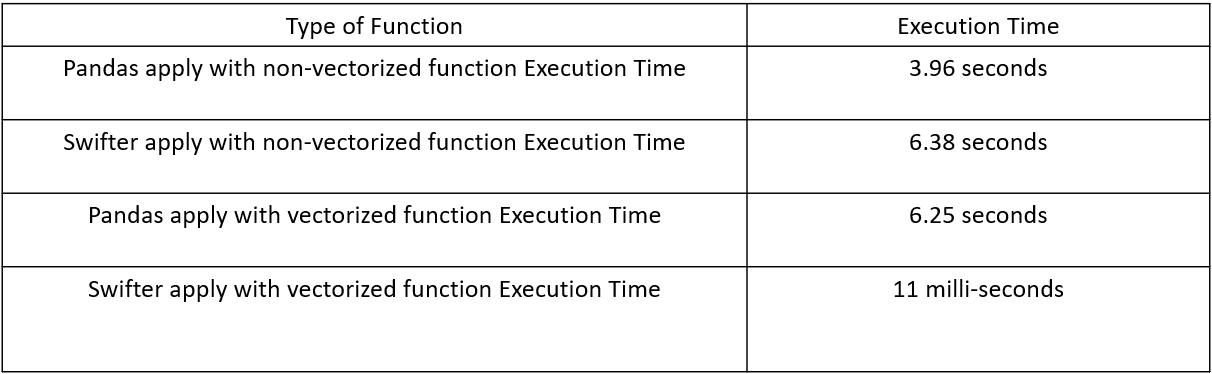#data-science #data-analysis #education #data analysis1656588180

## JSQCoreDataKit: A Swifter Core Data Stack

JSQCoreDataKit

A swifter Core Data stack

This library aims to do the following:

• Encode Core Data best practices, so you don't have to think "is this correct?" or "is this the right way to do this?"
• Provide better interoperability with Swift
• Harness Swift features and enforce Swift paradigms
• Bring functional paradigms to Core Data
• Make Core Data more Swifty
• Simplify the processes of standing up the Core Data stack
• Aid in testing your Core Data models
• Reduce the boilerplate involved with Core Data

• Xcode 13.0+
• Swift 5.5+
• iOS 11.0+
• macOS 10.12+
• tvOS 11.0+
• watchOS 4.0+
• SwiftLint

## Installation

### CocoaPods

``````pod 'JSQCoreDataKit', '~> 9.0.0'
``````

### Swift Package Manager

Add `JSQCoreDataKit` to the `dependencies` value of your `Package.swift`.

``````dependencies: [
.package(url: "https://github.com/jessesquires/JSQCoreDataKit.git", from: "9.0.0")
]
``````

Alternatively, you can add the package directly via Xcode.

## Documentation

You can read the documentation here. Generated with jazzy. Hosted by GitHub Pages.

## Contributing

Interested in making contributions to this project? Please review the guides below.

Also, consider sponsoring this project or buying my apps! ✌️

## Credits

Created and maintained by @jesse_squires.

Author: jessesquires
Source Code: https://github.com/jessesquires/JSQCoreDataKit

1658891012

## How to Increase Pandas Dataframe Rate with Swifter

Today we learn about Swifter, a Python module that allows us to speed up Pandas data frames by using multi-processing.

Swifter is a package that tries to efficiently apply any function to a Pandas Data Frame or Series object in the quickest available method. It is integrated with the Pandas object so that we would use this package only with a Pandas object such as Data Frame or Series.

📁 GitHub: https://github.com/NeuralNine

🎵 Outro Music From: https://www.bensound.com/1645144680

## swifter

A package which efficiently applies any function to a pandas dataframe or series in the fastest available manner.

## Documentation

To know about latest improvements, please check the changelog.

Further documentations on swifter is available here.

Check out the examples notebook, along with the speed benchmark notebook. The benchmarks are created using the library perfplot.

## Installation:

``````\$ pip install -U pandas # upgrade pandas
\$ pip install swifter # first time installation

``````

alternatively, to install on Anaconda:

``````conda install -c conda-forge swifter
``````

...after installing, import `swifter` into your code along with `pandas` using:

``````import pandas as pd
import swifter
``````

...alternatively, `swifter` can be used with `modin` dataframes in the same manner:

``````import modin.pandas as pd
import swifter
``````

NOTE: if you import swifter before modin, you will have to additionally register modin: `swifter.register_modin()`

## Easy to use

``````df = pd.DataFrame({'x': [1, 2, 3, 4], 'y': [5, 6, 7, 8]})

# runs on single core
df['x2'] = df['x'].apply(lambda x: x**2)
# runs on multiple cores
df['x2'] = df['x'].swifter.apply(lambda x: x**2)

# use swifter apply on whole dataframe
df['agg'] = df.swifter.apply(lambda x: x.sum() - x.min())

# use swifter apply on specific columns
df['outCol'] = df[['inCol1', 'inCol2']].swifter.apply(my_func)
df['outCol'] = df[['inCol1', 'inCol2', 'inCol3']].swifter.apply(my_func,
positional_arg, keyword_arg=keyword_argval)
``````

## Vectorizes your function, when possible## When vectorization is not possible, automatically decides which is faster: to use dask parallel processing or a simple pandas apply## Notes

1. The function is documented in the .py file. In Jupyter Notebooks, you can see the docs by pressing Shift+Tab(x3). Also, check out the complete documentation here along with the changelog.
2. Please upgrade your version of pandas, as the pandas extension api used in this module is a recent addition to pandas.
3. Import modin before importing swifter, if you wish to use modin with swifter. Otherwise, use `swifter.register_modin()` to access it.
4. Do not use swifter to apply a function that modifies external variables. Under the hood, swifter does sample applies to optimize performance. These sample applies will modify the external variable in addition to the final apply. Thus, you will end up with an erroneously modified external variable.
5. It is advised to disable the progress bar if calling swifter from a forked process as the progress bar may get confused between various multiprocessing modules.
6. If swifter return is different than pandas try explicitly casting type e.g.: `df.swifter.apply(lambda x: float(np.angle(x)))`